变量系列教材 （一）- Java中 什么是变量 变量系列教材 （三）- 什么是Java的字面值

Java中有八种基本类型，都是Java语言预先定义好的，并且是关键字。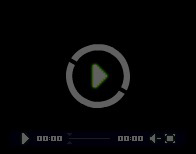9分23秒

## 整型

byte
short
int
long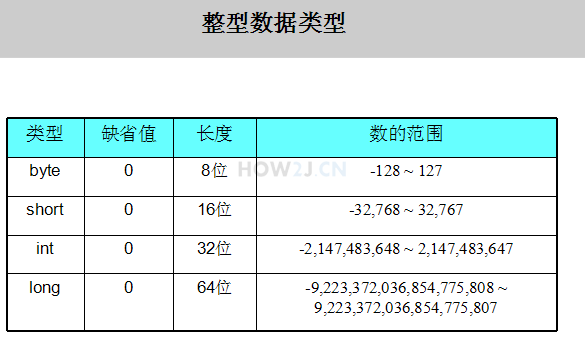public class HelloWorld{ public static void main(String[] args){ byte b = 1; short s = 200; int i = 300; long l = 400; /*如果试图给byte类型的变量赋予超出其范围的值，就会产生编译错误*/ byte b2 = 200; } }
```public class HelloWorld{

public static void main(String[] args){
byte b = 1;
short s = 200;
int i = 300;
long l = 400;

/*如果试图给byte类型的变量赋予超出其范围的值，就会产生编译错误*/
byte b2 = 200;
}
}
```

## 字符型

char类型用于存放一个字符，值用单引号'表示 (双引号表示字符串)

public class HelloWorld{ public static void main(String[] args){ char c = '中'; //char 只能存放一个字符，超过一个字符就会产生编译错误 char c2 = '中国'; //报错 char c3 = 'ab'; //报错 } }
```public class HelloWorld{

public static void main(String[] args){
char c = '中';
//char 只能存放一个字符，超过一个字符就会产生编译错误
char c2 = '中国'; //报错
char c3 = 'ab'; //报错

}
}
```

## 浮点型

float 长度为32位
double 长度为64位

float f2 = 54.321f,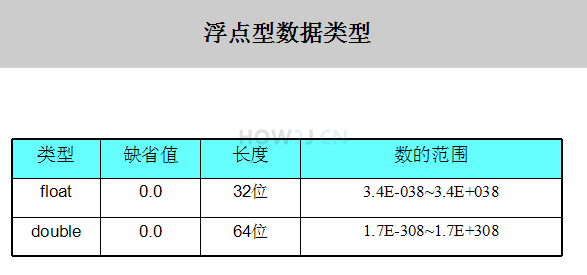public class HelloWorld{ public static void main(String[] args){ double d = 123.45; //该行会出现编译错误，因为54.321是double型的 float f = 54.321; float f2 = 54.321f; } }
```public class HelloWorld{

public static void main(String[] args){
double d = 123.45;

//该行会出现编译错误，因为54.321是double型的
float f = 54.321;

float f2 = 54.321f;

}
}
```

## 布尔型

boolean b1 = true;
boolean b2 = false;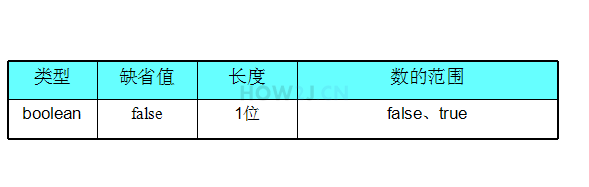public class HelloWorld { public static void main(String[] args) { boolean b1 = true; boolean b2 = false; // 虽然布尔型真正存放的数据是0(false) 1(true) // 但是，不能直接使用0 1 进行赋值 boolean b3 = 1; } }
```public class HelloWorld {

public static void main(String[] args) {

boolean b1 = true;
boolean b2 = false;

// 虽然布尔型真正存放的数据是0(false) 1(true)
// 但是，不能直接使用0 1 进行赋值
boolean b3 = 1;

}
}
```

## String类型

String类型其实并不是基本类型，但是它是如此广泛的被使用，常常被误以为是一种基本类型。
String类型是Immutable的，一旦创建就不能够被改变，更多的关于String的学习，请参考String
public class HelloWorld { public static void main(String[] args) { String str = "Hello Java"; } }
```public class HelloWorld {
public static void main(String[] args) {
String str = "Hello Java";
}
}
```

## 练习-变量类型

姿势不对,事倍功半! 点击查看做练习的正确姿势

3.14
2.769343
365
12
'吃'
false
"不可描述"

double a = 3.14;

## 答案-变量类型3分4秒 本视频采用html5方式播放，如无法正常播放，请将浏览器升级至最新版本，推荐火狐，chrome，360浏览器。 如果装有迅雷，播放视频呈现直接下载状态，请调整 迅雷系统设置-基本设置-启动-监视全部浏览器 (去掉这个选项)。 chrome 的 视频下载插件会影响播放，如 IDM 等，请关闭或者切换其他浏览器

public class HelloWorld { public static void main(String[] args) { double pi = 3.14; double e = 2.769343; short days = 365; byte months = 12; char chi = '吃'; boolean die = false; String fucking = "不可描述"; } }
```public class HelloWorld {
public static void main(String[] args) {
double pi = 3.14;
double e = 2.769343;
short days = 365;
byte months = 12;
char chi = '吃';
boolean die = false;
String fucking = "不可描述";
}
}
```

 变量系列教材 （一）- Java中 什么是变量 变量系列教材 （三）- 什么是Java的字面值

HOW2J公众号，关注后实时获知最新的教程和优惠活动，谢谢。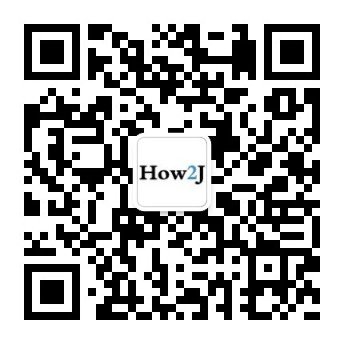2022-06-18 不理解字节的意思
 JohnnyJo 关于 JAVA 基础-变量-基本变量类型 的提问 我想问一下为什么365是用short；12用的是byte，我是初学者在我的理解中，byte是一个字节不是只能表达1个数字吗，short是2个字节不是只能表达2个数字吗？？ ```public class Test{ public static void main(String[]args) { double a = 3.14; double b = 2.769343; int c = 365; short d = 12; char f = '吃'; boolean e = false ; String g = "不可描述"; System.out.println(a); System.out.println(b); System.out.println(c); System.out.println(d); System.out.println(e); System.out.println(f); System.out.println(g); } }```

4 个答案

Rr2577588052

//整型变量用于存放整数 //byte -128~127 即-2^7 ---- 2^7-1 长度8 //short -32768~32767 即-2^15 ---- 2^15-1 长度16 //int -2147483648 - 2147483647 即-2^31 ---- 2^31-1 长度32 //long -9223372036854775808~9223372036854775807 即-2^63 ---- 2^63-1 长度64 // 这是我整理的

2021-12-29 关于float
 yolanda_hello_java 关于 JAVA 基础-变量-基本变量类型 的提问 为什么答案给出的 3.14 2.769343 都是double？ float的范围我的理解是包含这两个数的 用float可以吗？ 浮点数两个类型的范围是多大，表格中给的没明白

11 个答案

public class Variable { public static void main(String[]args){ double a =3.14; double a2 = 2.769343; short b = 365; byte c = 12; char d = "吃"; boolean e = false; String f = "不可描述"; } }

8866

0805_3

public static void main(String[] args) { double a = 3.14; float b = 2.769343f; int c = 365; byte d = 12; char e = '吃'; boolean f = false; String g = "不可描述"; }

fan541736130

char a = '樊'; double b=3.14; double c=2.769343; float d=2.769343f; short e=365; int q=365; long w=365l; byte r=12; char t ='吃'; boolean o=false; String name="不可描述";

fyc7015656

public class Item { float we = 3.14f ; double b = 2.769343 ; int x= 365 ; byte t = 12 ; char a= '吃'; boolean d=false ; String s= "不可描述" ; }

3.14 --> double 2.769343 --> double 365 --> int 12 --> int '吃' --> char false --> boolean "不可描述" --> String

Ljwstudying

Java中小数都默认是double类型的，如果想要用float类型表示，需要将进行类型转换，例如：float a = 3.14f;

Jn_L

float为单精度浮点类型，而double为双精度浮点类型，可以这样理解吧，如果小数点后只有一位可以用float型，如果后面有两位及以上就用double型；如果小数点后面又两位及以上你还想用float型，比如3.1415，这时你就要这样写 float a = 3.1415f

2021-10-22 代码报错
2021-09-25 浮点类型范围问题
2021-08-03 long类型的取值范围